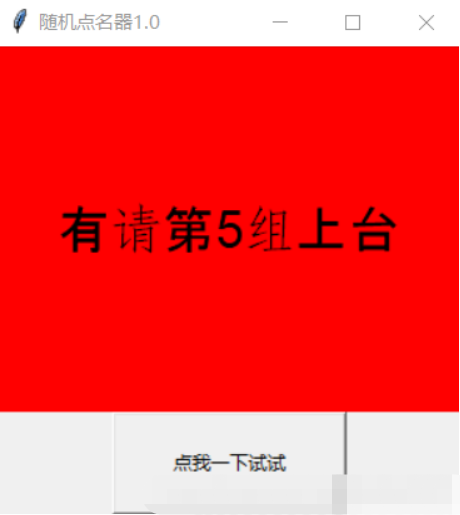# Python如何编写窗体程序• 月亮邮递员   2020-03-26 17:07:40435Python如何编写窗体程序

python和很多软件的用法其实差不多，只是代码更为简洁，代码量没这么多。

```import tkinter as tk
import random
bot = False
def func():
global bot
if bot==False:
bot=True
var.set("有请第%d组上台" % random.randint(1,14))
else:
bot=False
var.set('---------')
window = tk.Tk() #创建一个窗口对象
window.title("随机点名器1.0")#设置窗口标题
window.geometry('300x300')#设置窗口大小
var=tk.StringVar()#创建一个文字对象
l = tk.Label(textvar=var,font=('Arial',25),bg='red',width=40,height=6)#创建标签对象 设置文字内容，背景颜色，高和宽
l.pack()#放置按钮
but = tk.Button(text="点我一下试试",width=20,height=4,command=func)#创建按钮对象设置属性 command用来连接功能函数（方法）
but.pack()#放置按钮
window.mainloop()#运行```